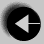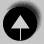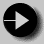# Functions in C

Almost all programming languages have some equivalent of the function. You may have met them under the alternative names subroutine or procedure.

Some languages distinguish between functions which return variables and those which don't. C assumes that every function will return a value. If the programmer wants a return value, this is achieved using the return statement. If no return value is required, none should be used when calling the function.

Here is a function which raises a double to the power of an unsigned, and returns the result.

```
double power(double val, unsigned pow)
{       double ret_val = 1.0;
unsigned i;

for(i = 0; i < pow; i++)
ret_val *= val;

return(ret_val);
}```

The function follows a simple algorithm, multiplying the value by itself pow times. A for loop is used to control the number of multiplications, and variable ret_val stores the value to be returned. Careful programming has ensured that the boundary condition is correct too. ie.

Let us examine the details of this function.

double power(double val, unsigned pow)
This line begins the function definition. It tells us the type of the return value, the name of the function, and a list of arguments used by the function. The arguments and their types are enclosed in brackets, each pair separated by commas.

The body of the function is bounded by a set of curly brackets. Any variables declared here will be treated as local unless specifically declared as static or extern types.

return(ret_val);
On reaching a return statement, control of the program returns to the calling function. The bracketed value is the value which is returned from the function. If the final closing curly bracket is reached before any return value, then the function will return automatically, any return value will then be meaningless.

The example function can be called by a line in another function which looks like this

```
result = power(val, pow);```

This calls the function power assigning the return value to variable result.

Here is an example of a function which does not return a value.

```
void error_line(int line)
{      fprintf(stderr, "Error in input data: line %d\n", line);
}```

The definition uses type void which is optional. It shows that no return value is used. Otherwise the function is much the same as the previous example, except that there is no return statement. Some void type functions might use return, but only to force an early exit from the function, and not to return any value. This is rather like using break to jump out of a loop.

This function also demonstrates a new feature.

fprintf(stderr, "Error in input data: line %d\n", line);
This is a variant on the printf statement, fprintf sends its output into a file. In this case, the file is stderr. stderr is a special UNIX file which serves as the channel for error messages. It is usually connected to the console of the computer system, so this is a good way to display error messages from your programs. Messages sent to stderr will appear on screen even if the normal output of the program has been redirected to a file or a printer.

The function would be called as follows

```
error_line(line_number);```Go to The goto StatementGo to IndexGo to Scope of Function